# 工程數學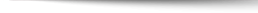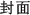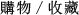## Advanced Engineering Mathematics 10/e Update (Taiwan Custom Version)

+作者：

### Kreyszig

+年份：
2018 年10 版
+ISBN：
9781119934165
+書號：
MA0458PCTS
+規格：

+頁數：
1152
+出版商：
John Wiley

\$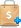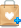• Parts of Chap. 1 on first-order ODEs are rewritten. More emphasis on modeling, also new block diagram explaining this concept in Sec. 1.1. Early introduction of Euler's method in Sec. 1.2 to familiarize student with basic numerics. More examples of separable ODEs in Sec. 1.3.
• For Chap. 2, on second-order ODEs, note the following changes: For ease of reading, the first part of Sec. 2.4, which deals with setting up the mass-spring system, has been rewritten; also some rewriting in Sec. 2.5 on the Euler-Cauchy equation.
• Substantially shortened Chap. 5, Series Solutions of ODEs. Special Functions: combined Secs. 5.1 and 5.2 into one section called "Power Series Method," shortened material in Sec. 5.4 Bessel's Equation (of the first kind), removed Sec. 5.7 (Sturm-Liouville Problems) and Sec. 5.8 (Orthogonal Eigenfunction Expansions) and moved material into Chap. 11 (see "Major Changes" above).
• New equivalent definition of basis (Sec. 7.4).
• In Sec. 7.9, completely new part on composition of linear transformations with two new examples. Also, more detailed explanation of the role of axioms, in connection with the definition of vector space.
• New table of orientation (opening of Chap. 8 "Linear Algebra: Matrix Eigenvalue Problems") where eigenvalue problems occur in the book. More intuitive explanation of what an eigenvalue is at the begining of Sec. 8.1.
• Better definition of cross product (in vector differential calculus) by properly identifying the degenerate case (in Sec. 9.3).
• Chap. 11 on Fourier Analysis extensively rearranged: Secs. 11.2 and 11.3 combined into one section (Sec. 11.2), old Sec. 11.4 on complex Fourier Series removed and new Secs. 11.5 (Sturm-Liouville Problems) and 11.6 (Orthogonal Series) put in (see "Major Changes" above). New problems (new!) in problem set 11.9 on discrete Fourier transform.
• New section 12.5 on modeling heat flow from a body in space by setting up the heat equation. Modeling PDEs is more difficult so we separated the modeling process from the solving process (in Sec. 12.6).
• Introduction to Numerics rewritten for greater clarity and better presentation; new Example 1 on how to round a number. Sec. 19.3 on interpolation shortened by removing the less important central difference formula and giving a reference instead.
• Large new footnote with historical details in Sec. 22.3, honoring George Dantzig, the inventor of the simplex method.
• Traveling salesman problem now described better as a "difficult" problem, typical of combinatorial optimization (in Sec. 23,2]." More careful explanation on how to compute the capacity of a cut set in Sec. 23.6 (Flows on Networks).
• Additional problems for Chapters '16-19, 22 and 23 are available on line at www.wiley.com/go/global/kreyszig.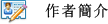Erwin Kreyszig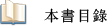PART A Ordinary Differential Equations (ODEs)
CHAPTER 1 First-Order ODEs
CHAPTER 2 Second-Order Linear ODEs
CHAPTER 3 Higher Order Linear ODEs
CHAPTER 4 Systems of ODEs. Phase Plane. Qualitative Methods
CHAPTER 5 Series Solutions of ODEs. Special Functions
CHAPTER 6 Laplace Transforms
PART B Linear Algebra. Vector Calculus
CHAPTER 7 Linear Algebra: Matrices, Vectors, Determinants. Linear Systems
CHAPTER 8 Linear Algebra: Matrix Eigenvalue Problems
CHAPTER 9 Vector Differential Calculus. Grad, Div, Curl
CHAPTER 10 Vector Integral Calculus. Integral Theorems
PART C Fourier Analysis. Partial Differential Equations (PDEs)
CHAPTER 11 Fourier Analysis
CHAPTER 12 Partial Differential Equations (PDEs)
PART D Complex Analysis
CHAPTER 13 Complex Numbers and Functions. Complex Differentiaion
CHAPTER 14 Complex Integration
CHAPTER 15 Power Series, Taylor Series
CHAPTER 16 Laurent Series. Residue Integration
CHAPTER 17 Conformal Mapping
CHAPTER 18 Complex Analysis and Potential Theory
PART E Numeric Analysis
Software
CHAPTER 19 Numerics in General
CHAPTER 20 Numeric Linear Algebra
CHAPTER 21 Numerics for ODEs and PDEs
PART F Optimization, Graphs
CHAPTER 22 Unconstrained Optimization. Linear Programming
CHAPTER 23 Graphs. Combinatorial Optimization